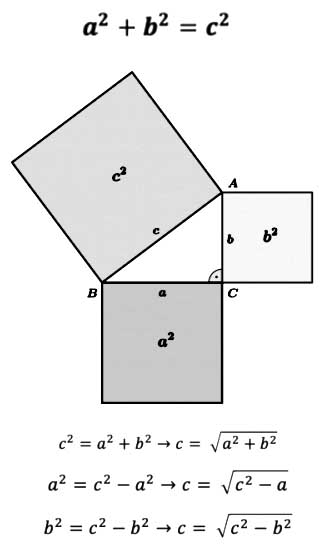# Pythagorean theorem – Calculator

Pythagorean theorem – CalculatorIn any right triangle, the sum of the squares of the lengths of the legs is equal to the square of the length of the hypotenuse of the triangle. As indicated in the figure, there is identity.

Geometrically it means, that if we build squares on the sides of a right triangle, is the sum of the areas of squares built on the hypotenuse of this triangle will be equal to the area of ​​the square built on the hypotenuse.

Choose, do you want to find the value of A, B or C and enter the other two values!$a^{2}+b^{2}=c^{2}\Leftrightarrow c= \sqrt{a^{2}+b^{2}}$